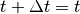# Trouble Shooting¶

I found a bug or have a feature request. How can I get in touch ?
Please post issues here or via email mjhoffmann .at. gmail .dot. com or via twitter @maxjhoffmann
My rate constant expression doesn’t work. How can I debug it?

When initializing the model, the backend uses kmos.evaluate_rate_expression. So you can try

```from kmos import evaluate_rate_expression
evaluate_rate_expression('<your-string-here'>, parameters={})
```

where parameters is a dictionary defining the variable that are defined in the context of the expression evaluation, like so

```parameters = {'T': {'value': 500},
'p_NClgas': {'value': 1},
}
```

Test only parts of your expression to localize the error. Typical mistakes are syntax errors (e.g. unclosed parentheses) and forgotten conversion factors (e.g. eV) which can easily lead to overflow if written in the exponent.

How can I print the chemical potential value, that kmos is using internally?

You can then print the explicit value for specific conditions in kmos shell, for example like so

```from kmos import evaluate_rate_expression
print(
evaluate_rate_expression('mu_COgas',
{'T':{'value':600},
'p_COgas': {'value':1}
}
)))
```

where ‘CO’ should be replaced by whatever gas species you are inspecting. And the resulting number is given in eV. kmos linearly interpolates the gas phase chemical potential from the NIST JANAF thermochemical tables if you have downloaded them manually. If you don’t have them installed, an error message should get raised which explains how to do so.

When I use kmos shell the model doesn’t have the species and sites I have defined.
Note that Fortran is case-insensitive. Therefore f2py turns all variable and functions names into lower case by convention. Try to lower-case your species or site name.
When I run kmos the GUI way and close it, it seems to hang and I need to use the window manager to kill it.
This is a bug waiting to be fixed. To avoid it close the window showing the atoms object by clicking on its close button or Alt-F4 or whichever shortcut your WM uses.
Running a model it sometimes prints Warning: numerical precision too low, to resolve time-steps

This means that the kMC step of the current process was so small compared to the current kMC time that for the processor. This should under normal circumstances only occur if you changed external conditions during a kMC run.

Otherwise it could mean that your rate constants vary over 12 or more orders of magnitude. If this is the case one needs to wonder whether non-coarse graind kMC is actually the right approach for the system. On the hand because the selection of the next process will no longer be reliable and second because reasonable sampling of all involved process may no longer happen.

When running a model without GUI evaluation steps seem very slow.
If you have a kmos.run.KMC_Model instance and call model.get_atoms() the generation of the real-space geometry takes the longest time. If you only have to evaluate coverages or turn-over frequencies you are better off using model.get_atoms(geometry=False), which returns an object with all numbers but without the actual geometry.
What units is kmos using ?
By default length are measured in angstrom, energies in eV, pressure in bar, constants are taken from CODATA 2010. Note that the rate expressions though contain explicit conversion factors like bar, eV etc. If in doubt check the resulting rate constants by hand.
How can I change the occupation of a model at runtime?

This is explained in detail at Manipulating the Model at Runtime though the import bit is that you call

```model._adjust_database()
```

after changing the occupation and before doing the next kMC step.

How can I quickly obtain k_tot ?
That is ::
model.base.get_accum_rate(model.proclist.nr_of_proc)
How can I check the system size ?
You can check ::
model.lattice.system_size

to get the number of unit cell in the x, y, and z direction. The number of sites per unit cell is stored in

```model.lattice.spuck
```

a.k.a Sites Per Unit Cell Konstant :-). Or you check

```model.base.get_volume()
```
to get the total number of sites, i.e. ::
model.base.get_volume() == model.lattice.system_size.prod()*model.lattice.spuck => True

More to follow.

Todo

Explain post-mortem procedure